Editor Ratings:
User Ratings:
[Total: 3 Average: 3]

Here is a list of 10 Free Online Calculator with steps. These free online calculators can help you see each step of the calculation. You just need to enter your unsolved math expressions and these calculators solve the calculations in seconds. Some of the sites let you print the calculation while some sites let you generate a PDF. In this way, you can understand Math subject looking at each step.

Solving math expressions and equations online is easy. But sometimes what you calculate, you don’t get the steps of your calculations. Using these online calculator with steps help to give you an explanation that how the calculator reached the answer. In this way, you can check and understand how the sum is being solved. Also, you don’t need to sign up for each website. But if you wish to sign up then it is free.

Let’s understand how these online calculators work with steps.

## 1. Symbolab

Symbolab is a good website that lets you solve math expression and other calculations easily. It solves equations from easy to hard. Also, it shows steps of your calculations. You just need to add your problem in the search bar and it will solve it with steps. The site solves all kind of math problems which include Algebra, matrices, geometry, trigonometry, etc.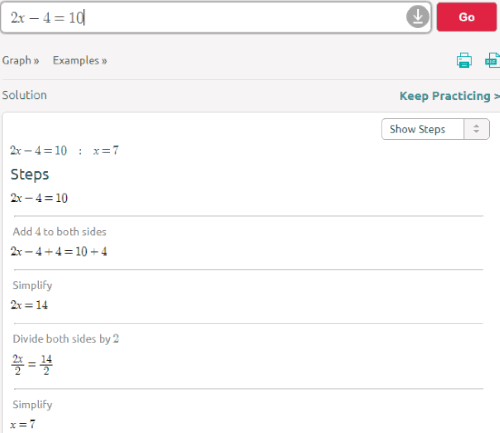Once you open the site, you will see a search bar in which you can enter your problem. After entering your calculation you can click on the Go button. Thereafter, it will show you steps of your calculation. As you can see in the above image, I entered an equation (2x-4=10) in the search bar and clicked on Go. Then it showed me steps of how the equation was solved.

You can also take out prints or can generate a PDF of your calculation. In this way, you can prepare some examples of math problems on a sheet of paper. Thereafter, you can keep those papers as notes for math practice.

Using this website, you can also practice physics and chemistry subjects. You can calculate physics formulas and chemical reactions with steps. Also, the site offers you 6 different languages. Default language is set to English.

## 2. MathPapa

MathPapa is another website of an online calculator with steps. The site is a good Algebra calculator that can help a student in practicing math subject easily. It shows each step of your calculation and a student can check those steps in order to understand the equation.You can see in the above image, here, I entered a simple equation in the search bar and clicked on calculate it. Thereafter, the site solved my equation in seconds. One thing to note is that when you enter the small calculation, the site will show the steps automatically but for the big calculations like {4x+2=2(x+6)} you need to click on show step by step. Thereafter, it will show you each step of your calculation.

Using this calculator, you can solve math equations easily. The site also lets you practice algebra. To do practice, you can click on the practice button that appears on the homepage next to the algebra calculator. The practice includes basic arithmetic, basic equations, fractions, percentage, etc., where you can fill the answers in order to test yourself.

Though you can do calculations without signing up. But if you sign up you can see your previous calculations done on Mathpapa.

You can also subscribe to their YouTube channel to watch videos of solving sums.

## 3. cymath

cymath is an online calculator with steps. Using this calculator, you can choose the topic by clicking on the topic. The drop down will show you a list of many topics, you can select one and then start calculating.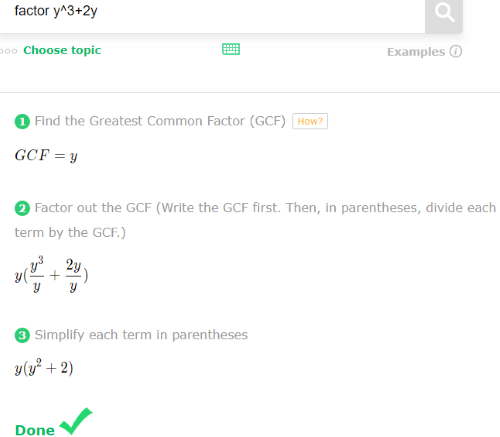In the above image, I selected a topic of factor. Then I entered my equation (y 3 +2y) in the bar. This calculator solved my sum in seconds. Once you enter your problem in the search bar, it will solve your equation and will show you each step of solving the calculation. In this way, you can understand other math topics also by using this calculator.

You can also practice math expression with different topics. This calculator is available in 5 different languages like English, Japanese, etc. There is no need to sign up to solve the calculation. You can just visit the site and get the steps of your calculations.

## 4. QuickMath

QuickMath is a simple online calculator with steps. You can enter your calculations in the search bar then you can get the solved equation with each step. It solves math expression quickly as the name suggests QuickMath. The site offers different math topics like Algebra, Equations, Graphs, Numbers, etc. You can also change the levels of solving math from simple to advanced. You can also learn the definition of any topic by clicking on the topic name.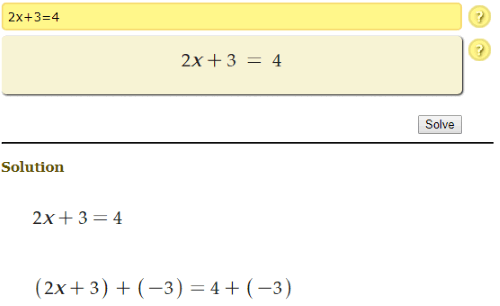Once you visit the site, you can enter your equation and it gets solved in a few seconds. You can scroll the page to see the steps of your sum. The site also offers a tutorial. However, the tutorial page is in progress but later you can get advanced education of practicing math.

## 5. WolframAlpha

WolframAlpha is a good online calculator with steps. Using this calculator, you can see the steps to understand the calculation. Also, it lets you see the graph of your equation. You can just enter your calculation and then it will show you an explanation with steps.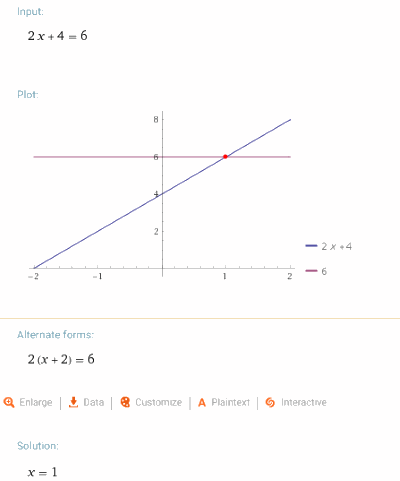As you can see in the above image, I entered my equation and then it showed these steps. Firstly it showed a plot and an alternate form and the solution. You can also save your calculation as favorite. It lets you see the history also. However, you cannot do this without signing up.

## 6. Hackmath.net

This (Hackmath.net) is another online calculator with steps. The site has a very simple format to answer the calculation. You can just enter your equation and then the online calculator will answer the question.In the above image, you can see I entered an unsolved LCM and then I clicked on Calculate LCM. Thereafter, it instantly solved it. You can see the steps below. It shows each step how the LCM is being solved.

You can calculate other topics of math like algebra, fraction, LCM, decimals and so on. This online calculator with steps can help you do calculations easily in less time.

## 7. wyzant.com

This (wyzant.com) is another online calculator with steps where you can enter your equation and set variables and then click on solve. Thereafter, the calculator will show you the steps of the equation and how it was being solved.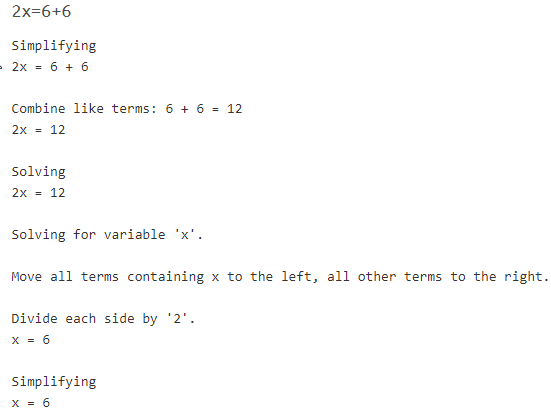You can see in the above image, here I entered a simple equation and then it solved it in seconds. You can take lessons and worksheets for math practice. Also, you can choose the topic and then you can solve math expression in a few seconds. This is for all levels of students.

## 8. Mathportal.org

Mathportal.org is an online calculator with steps. Using this calculator, you can get your equation solved. Also, you see the steps of your calculations. The site offers other math lessons also. You can share a direct link of any calculation that you make. The site also lets you generate a PDF of your calculation.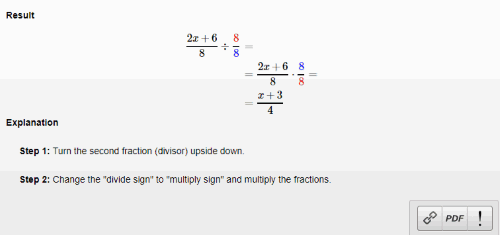The above image is simply showing you steps. Here I entered the equation and then each step and its explanation is mentioned below the picture.

There are other lessons that you can learn on the site. For example, polynomial, radical expression, equations, etc. This calculator can help you to do calculations easily.

## 9. Calculator soup

This calculator soup is an online calculator with steps. It helps you see the calculations step by step. The site offers you different kinds of lessons like a fraction, statistics, basic algebra, geometry, etc.In the above image, you can see each step is shown below the equation I entered. Here, everyone can understand the steps of the calculations.

Calculator soup also works for Math, Physics, Chemistry. You can use this calculator to see steps for calculation.

## 10. Integral Calculator

Integral calculator is an online calculator with steps. Using this calculator, you can solve your math equations and then check each step in order to understand the calculation.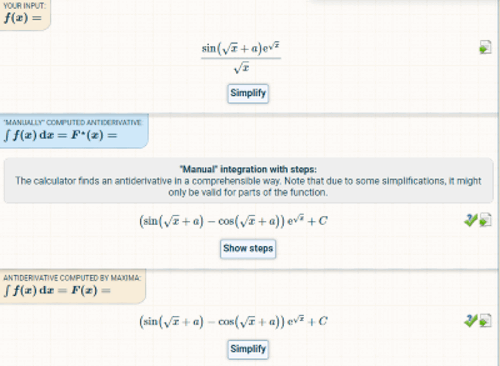In the above image, you can see how it is showing each step of the equation I entered to calculate. The site shows you step by step calculation. It also supports definite and indefinite integral with variables. It is easy to use.